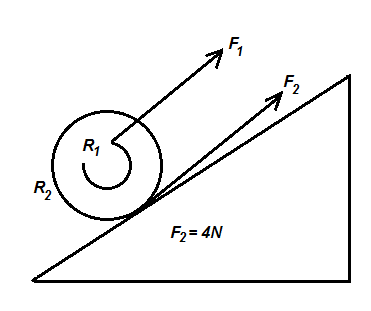Courses
Courses for Kids
Free study material
Offline Centres
MoreLast updated date: 02nd Dec 2023
Total views: 344.4k
Views today: 8.44k

# A bicycle can go up a gentle inclination with constant speed where the frictional force of ground pushing the rear wheel is ${F_2} = 4\,{\text{N}}$ . With what force ${F_1}$ must the chain pull on the socket wheel if ${R_1} = 4\,{\text{cm}}$ and ${R_2} = 30\,{\text{cm}}$ ?A. $4\,{\text{N}}$B. $24\,{\text{N}}$C. $140\,{\text{N}}$D. $\dfrac{{35}}{4}\,{\text{N}}$Verified
344.4k+ views
Hint: First of all, we will find the individual torques for the two different forces. After that we will equate both the equations as the net torque is zero for a body moving at constant speed. We will manipulate accordingly and obtain the result.

In the given problem, we are supplied with the following data:
The frictional force of ground pushing the rear wheel is the frictional force of ground pushing the rear wheel is $4\,{\text{N}}$ .
The radius of the rear socket wheel is $30\,{\text{cm}}$ .
The radius of the front socket wheel is $4\,{\text{cm}}$ .
We are asked to find the force ${F_1}$ , with which the chain pulls on the socket wheel.

To begin with, we have to know that this problem is based on the rolling motion. Again, we can observe that the bicycle is moving up the inclined plane with a speed which is constant. This can be used to use the net force and the net torque is also zero.
First case:
The torque developed due to the force ${F_1}$ can be expressed with the equation:
${\tau _1} = {r_1} \times {F_1}$ …… (1)
Where,
${\tau _1}$ indicates the torque developed by the force.
${r_1}$ indicates the radius of the smaller socket wheel.
${F_1}$ indicates the force with which the chain pulls on the socket wheel.

Second case:
The torque developed due to the force ${F_2}$ can be expressed with the equation:
${\tau _2} = {r_2} \times {F_2}$ …… (2)
Where,
${\tau _2}$ indicates the torque developed by the force.
${r_2}$ indicates the radius of the smaller socket wheel.
${F_2}$ indicates the frictional force.

Since, the net torque is zero, we can write:
${\tau _1} = {\tau _2} \\ {r_1} \times {F_1} = {r_2} \times {F_2} \\$
${F_1} = \dfrac{{{r_2} \times {F_2}}}{{{r_1}}}$ …… (3)

Substituting the required values in the equation (3), we get:
${F_1} = \dfrac{{30 \times 4}}{5} \\ {F_1} = 24\,{\text{N}} \\$
Hence, the force with which the chain pulls on the socket wheel is $24\,{\text{N}}$ .
The correct option is B.

Note:It is important to note that if a rotatable object has a net torque of zero, it will be in rotational equilibrium and will not be able to obtain angular acceleration. Torque is therefore zero when the body is static.